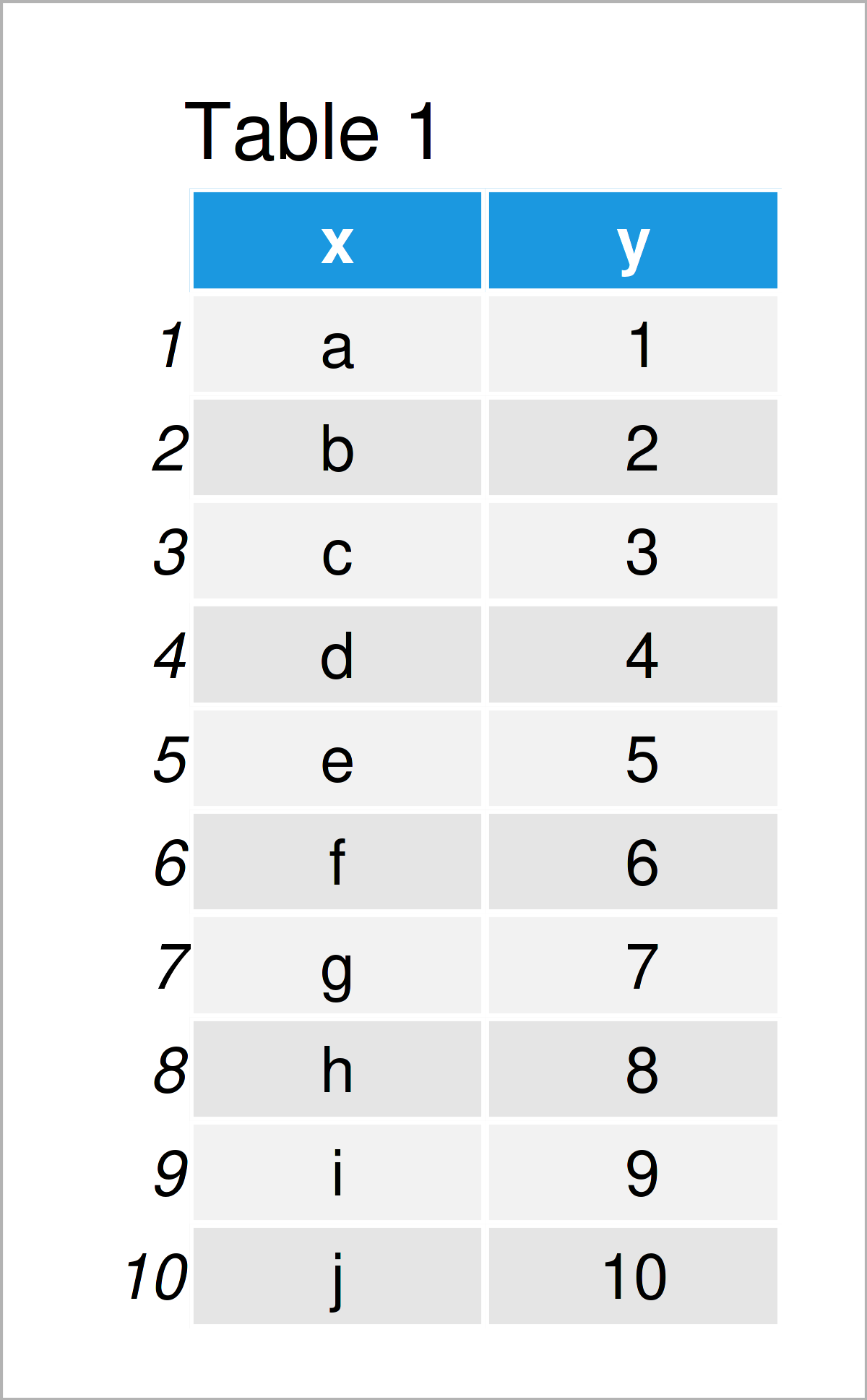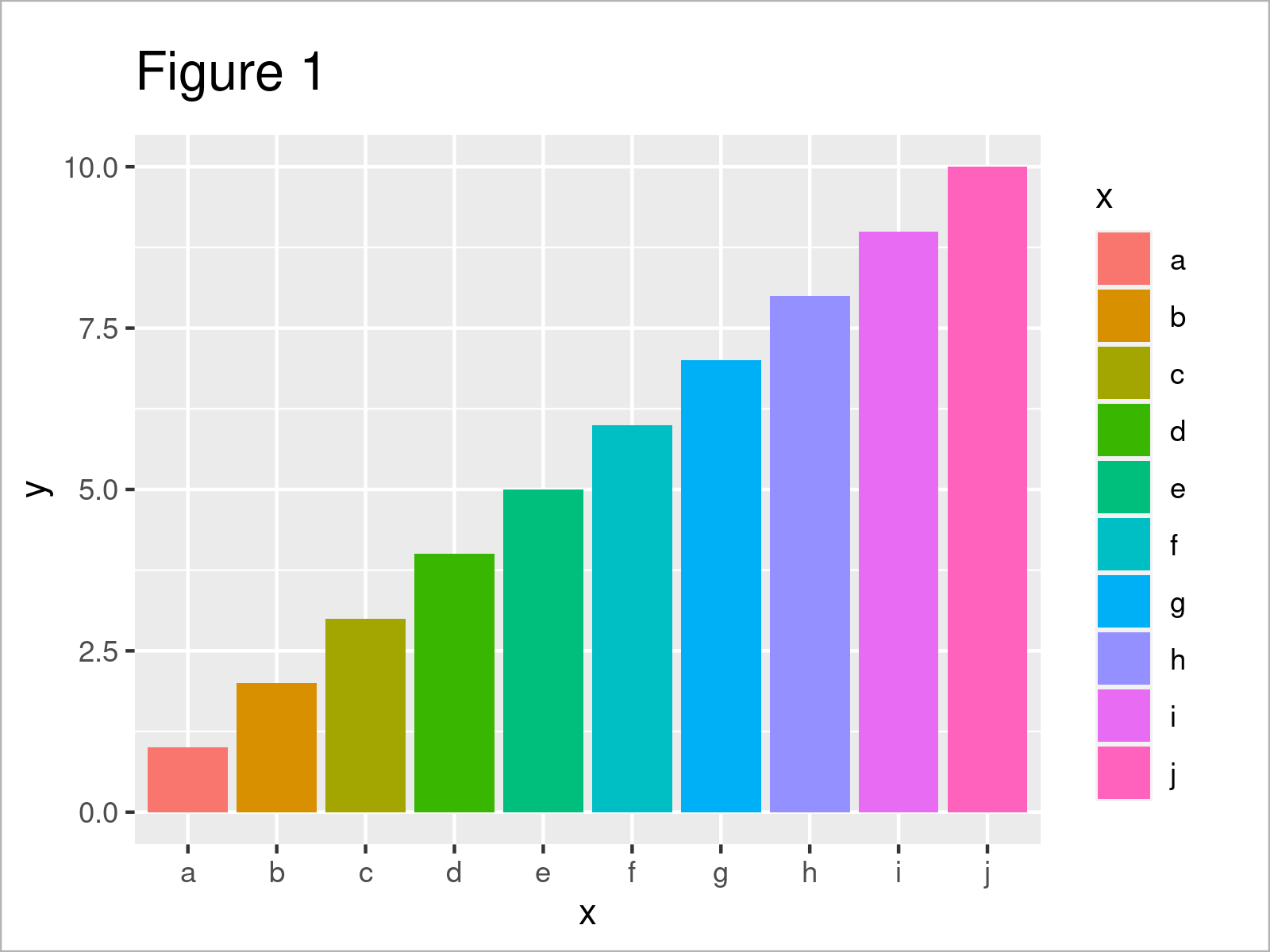# Draw ggplot2 Plot with Grayscale in R (2 Examples)

In this article, I’ll explain how to create a ggplot2 plot with grayscale in R.

The content is structured as follows:

Let’s get started.

## Example Data, Software Packages & Default Graphic

The following data is used as basement for this R tutorial:

```data <- data.frame(x = letters[1:10], # Create example data y = 1:10) data # Print example data```Table 1 illustrates the structure of our example data: It has ten rows and the two variables “x” and “y”.

For the following tutorial, I’ll also have to install and load the ggplot2 package:

```install.packages("ggplot2") # Install & load ggplot2 package library("ggplot2")```

Now, we can create a graph of our data as follows:

```ggp <- ggplot(data, aes(x, y, fill = x)) + # Create default ggplot2 plot geom_bar(stat = "identity") ggp # Draw default ggplot2 plot```The output of the previous R syntax is shown in Figure 1 – A ggplot2 barplot with multiple different colors.

## Example 1: Convert ggplot2 Plot to Grayscale Using scale_fill_grey() Function

In Example 1, I’ll illustrate how to draw a ggplot2 graphic in grayscale.

For this task, we simply have to add the scale_fill_grey function to our plot:

```ggp + # Convert ggplot2 plot to grayscale scale_fill_grey()```In Figure 2 you can see that we have created a new version of our plot in which all colors have been converted to a gray color range.

This is typically a great look for research publications in scientific journals, since many journals do not provide color prints.

However, there’s even more we can improve!

## Example 2: ggplot2 Plot with Grayscale & Simple Theme

In this example, I’ll illustrate how to simplify the theme of our plot to match it better to the grayscale color range.

A very commonly used ggplot2 theme is the theme_bw (i.e. theme black & white).

We can use this theme as shown below:

```ggp + # Grayscale & simple theme scale_fill_grey() + theme_bw()```As shown in Figure 3, the previous R programming code has created a new version of our barplot with simplified black and white background.

## Video, Further Resources & Summary

Would you like to learn more about the creation of a ggplot2 graphic with gray colors? Then you might want to watch the following video on my YouTube channel. In the video, I illustrate the R programming syntax of this page in RStudio.

Please accept YouTube cookies to play this video. By accepting you will be accessing content from YouTube, a service provided by an external third party.If you accept this notice, your choice will be saved and the page will refresh.

Furthermore, you may want to have a look at some of the related articles on my website. I have published numerous tutorials on topics such as dates, time objects, graphics in R, and lines: